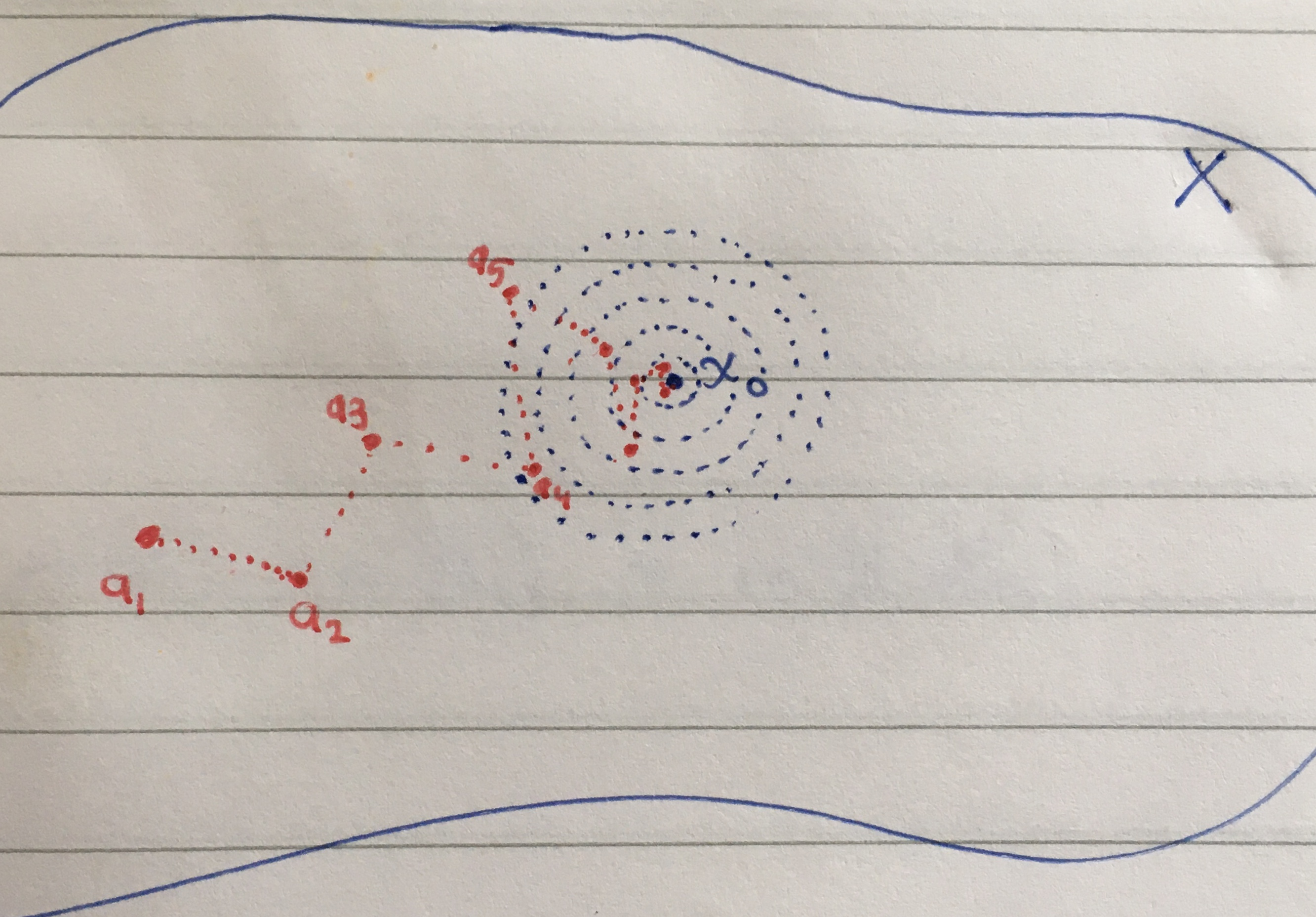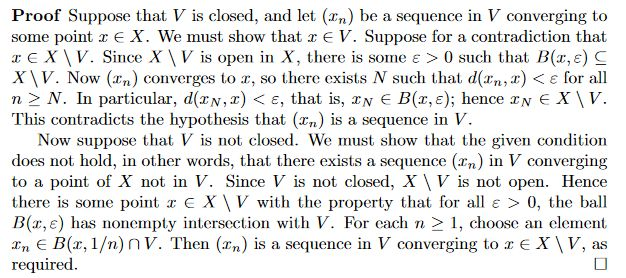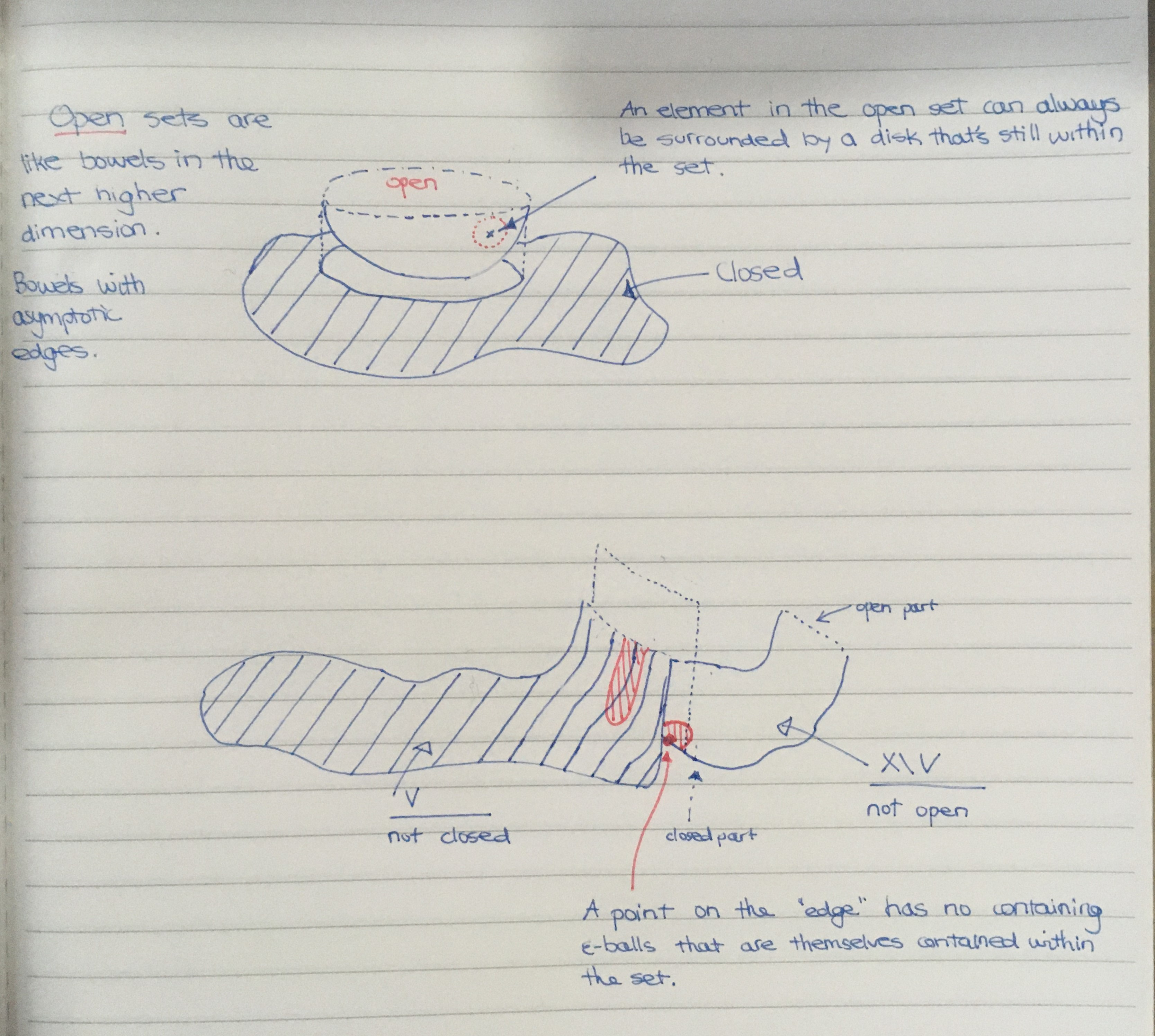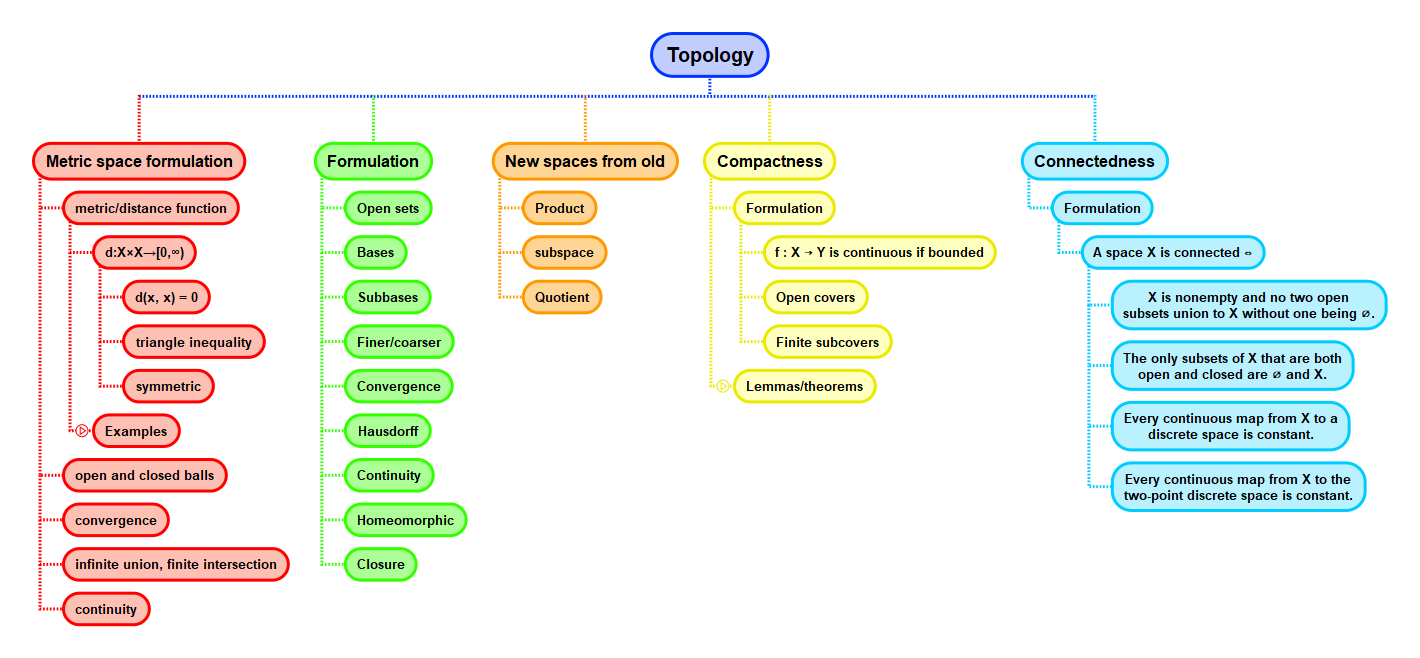Show Question
Math and science::Topology

# Metric space. Sequence convergence

### Sequence convergence, definition

Let $$X$$ be a metric space, let $$x_0 \in X$$ and let $$(a_n)_{n=1}^{\infty}$$ be a sequence in $$X$$.

$$(a_n)_{n=1}^{\infty}$$ is said to converge to $$x_0$$ iff

$$\lim_{n \to \infty} d(x_0, a_n) = 0$$

Unfolding the definition of a limit this becomes: $$(a_n)_{n=1}^{\infty}$$ converges to $$x_0$$ iff for any $$\varepsilon > 0$$ there exists an $$N \ge 1$$ such that for all $$i \ge N$$, $$d(x_0, a_i) < \varepsilon$$.This next result should be internalized

### Lemma. Closed iff sequences converge inside

Let $$X$$ be a metric space and $$V \subseteq X$$.

Then $$V$$ is closed in $$X$$ $$\iff$$ for all sequences $$(x_n)$$ in $$V$$ and all $$x$$ in $$X$$, if $$(x_n)$$ converges to $$x$$ then $$x \in X$$.

The proof was not immediately obvious to me. I did, however, find a way to visualize it, which I think makes the line of reasoning clear. Part of why the proof is important, I think, is that there are only two notions available from which the result needs to be derived: the definition of open sets and the definition of sequence convergence. So, it should be simple, yet it escapes an immediately obvious proof. Thus, I feel there is a mode of thinking that has its essence somewhat distilled into the line of reasoning.The forward part of the proof corresponds to the idea that if you choose any point not within $$V$$, then it will be in an open set, and thus, there is always some ε-ball around the point that is fully contained within the enclosing open set.

The reverse part of the proof corresponds to the idea that if $$V$$ is not closed, then $$X \setminus V$$ is not open, so that, somewhere on the boundary between $$V$$ and $$X \setminus V$$, there must be a "hard" edge on the $$X \setminus V$$ side of the boundary and a "soft"/open edge on the $$V$$ side. Because of this, any ε-ball around this point must spill into $$V$$. By choosing successively smaller ε-balls, we can make a sequence in $$V$$ that converges to the point in $$X \setminus V$$.## Context